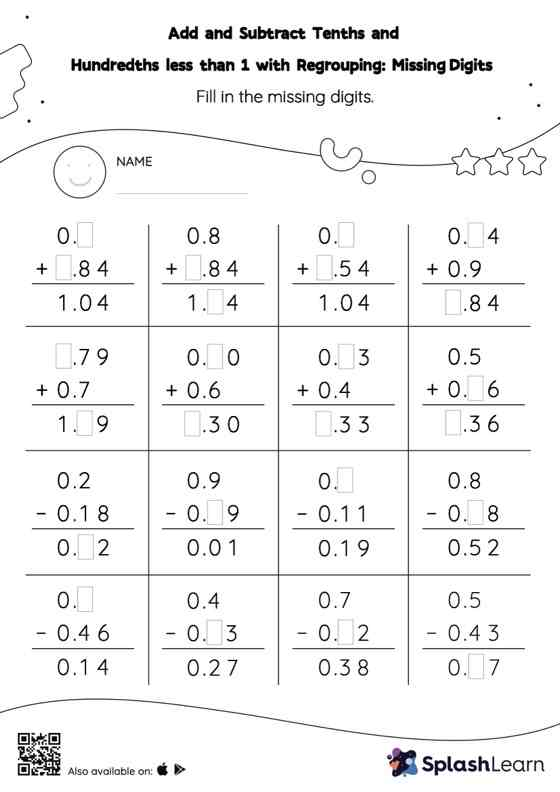# Add and Subtract Tenths and Hundredths less than 1 with Regrouping: Missing Digits Worksheet

Home > Add and Subtract Tenths and Hundredths less than 1 with Regrouping: Missing DigitsAdd and subtract tenths and hundredths less than 1 with regrouping worksheet helps students develop proficiency with addition and subtraction. Students find the missing number while adding or subtracting decimals by using the relationship between addition and subtraction. To reach the answer, they also use the relationship between ones, tenths, hundredths, thousandths, etc., To regroup the numbers in add and subtract tenths and hundredths less than 1 with regrouping worksheet. As the worksheet uses the column method, it is helpful in getting students toward higher accuracy, especially with bigger numbers and in scenarios where regrouping is required.